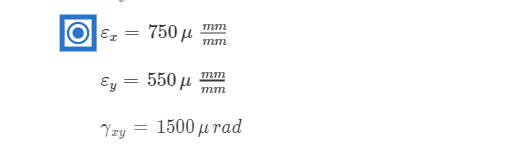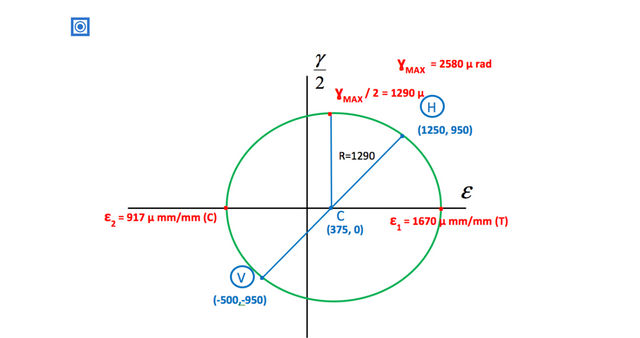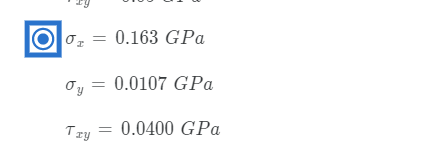Mechanics of Materials Fundamentals of Stress & Strain and Axial Loading Coursera All Weeks Quiz

Week- 1

Quiz on Stress and Strain Fundamentals

1. For the truss shown below, member BC is a circular solid bar with a 25 mm diameter.

Find:

(a) the normal and shear stress in member BC on a non-transverse plane 35° from the vertical as shown

(b) the maximum normal stress and maximum shear stress in member BC

• On non-transverse cut: \sigma =55.0\,MPa\left( T \right)\,\,\,\,\tau =38.5\,MPa\,\,\,\,σ=55.0MPa(T)τ=38.5MPa Max Values: {{\sigma }_{MAX}}=82.0\,MPa\left( T \right)\,\,\,\,{{\tau }_{MAX}}=41.0\,MPa\,\,\,\,σ
MAX? =82.0MPa(T)τ MAX =41.0MPa
• On non-transverse cut: σ=75.0MPa(T)τ=50.0MPa Max Values: σMAX=125MPa(T)τMAX=62.5MPa
• On non-transverse cut: σ=67.3MPa(T)τ=47.1MPa Max Values: σMAX=100MPa(T)τMAX=50.1MPa
• None of the above

2. For the engineering structure below you may assume the weight of the members to be negligible when compared to the loads they are supporting. All pin connections may be considered frictionless. A portion of member JH is a hollow steel tube with an inner radius of 10mm and an outer radius of 13 mm. The normal stress for a transverse cut through this member is 200 MPa in compression. Find the axial force in member JH.

• The axial force in member JH is 1.88 kN in compression.
• The axial force in member JH is 10.9 kN in compression.
• The axial force in member JH is 43.4 kN in compression.
• None of the above

3. For the engineering structure below you may assume the weight of the members to be negligible when compared to the loads they are supporting. All pin connections may be considered frictionless.

Bar DE is aluminum and has a cross sectional area of 5000 m{{m}^{^{2}}}mm
2

and has a normal stress of 200 MPa in compression.

Bar BC is steel and has a cross sectional area of 1300 m{{m}^{^{2}}}mm
2

and has a normal stress of 150 MPa in tension.

Find the force P

• P is 352 kN to the right
• P is 556 kN to the right
• P is 878 kN to the right
• None of the above

Week- 2

Quiz on Stress-Strain Diagrams, Material Properties, and Shear Stress and Strain

1. A circular section of material is tested. The original specimen is 200 mm long and has a diameter of 13 mm. When loaded to its proportional limit, the specimen elongates by 0.3 mm. The total axial load is 20 kN. Determine the modulus of elasticity and the proportional limit.

• a) Modulus of Elasticity: E = 100 GPa Proportional Limit: {{\sigma }_{Proportional Limit }}=0.151\,GPa\,\,σ Proportional Limit=0.151GPa
• Modulus of Elasticity: E = 50 GPa Proportional Limit: {{\sigma }_{Proportional Limit }}=0.075\,GPa\,\,σ
Proportional Limit =0.075GPa
• Modulus of Elasticity: E = 150 GPa Proportional Limit: {{\sigma }_{Proportional Limit }}=0.225\,GPa\,\,σ
Proportional Limit =0.225GPa
• None of the above

2. A rectangular test section of material is slowly loaded with a 450 kN axial load. The original cross-section is 25 mm by 100 mm. The original length is 2 meters. Once fully loaded, the 100 mm side measures 99.96 mm and the length has increased by 2 mm. Calculate Poisson’s ratio and Young’s modulus.

• a) Poisson’s Ratio: \nuν = 0.4 Young’s Modulus: E = 180 GPa
• Poisson’s Ratio: \nuν = 0.25 Young’s Modulus: E = 120 GPa
• Poisson’s Ratio: \nuν = 0.6 Young’s Modulus: E = 200 GPa
• None of the above

3. A thin rectangular plate section of an airplane is uniformly deformed as shown below. (We will learn how to measure strains later in the course.) Compute the shear strain at point A. The original sides AB and AC were orthogonal in the undeformed plate.

• The shear strain at point A is 1500 µ rad
• The shear strain at point A is 1670 µ rad
• The shear strain at point A is 95500 µ rad
• None of the above

Week- 3

Quiz on Stresses on Inclined Planes

1. Use the plane stress transformation equations to find the normal stress and the magnitude of the shear stress on plane A-A for the stress acting at a point in a machine component shown below.

• The normal stress on plane AA is 0.836 MPa (T) and the magnitude of the shear stress on plane AA is 110 MPa
• The normal stress on plane AA is 50 MPa (T) and the magnitude of the shear stress on plane AA is 90 MPa
• The normal stress on plane AA is 67.5 MPa (T) and the magnitude of the shear stress on plane AA is 72.7 MPa
• None of the above

2. Find the principal stresses and the maximum in-plane shear stress for the stress condition acting at a point in a machine component shown below.

• The principal stresses are 129 MPa (T) and 94.4 MPa (C). The maximum in-plane shear stress is 112 MPa.
• The principal stresses are 94.4 MPa (T) and 129 MPa (C). The maximum in-plane shear stress is 112 MPa.
• The principal stresses are 100 MPa (T) and 150 MPa (C). The maximum in-plane shear stress is 125 MPa.
• None of the above

3. Show a Mohr’s Circle plot depicting the principal stresses and the maximum in-plane shear stress for the stress condition acting at a point in an engineering structural member shown below.Week- 4

Quiz on Stress concentrations, Mohr’s Circle for Plane Strain, and measuring strains

1. For an engineering structural member under loading, the strain components at a point are found to be{{\varepsilon}_{x}}=\,-500\,\mu\,\frac{mm}{mm}ε x? =−500μ mmmm? {{\varepsilon }_{y}}=\,1250\,\mu \,\frac{mm}{mm}ε y? =1250μmmmm{\gamma }_{xy}}=\,1900\,\mu \,radγ xy? =1900μradFind the strain components {{\varepsilon }_{n}}ε n? and {{\gamma}_{nt}}γ nt?
for the n-t axes oriented as shown below:

• {{\varepsilon }_{n}}=\,415\,\mu \,\frac{mm}{mm}ε n? =415μ mmmm? and {{\gamma }_{nt}}=\,2560\,\mu \,radγ nt? =2560μrad
• {{\varepsilon }_{n}}=\,-915\,\mu \,\frac{mm}{mm}ε n? =−915μ mmmm? and {{\gamma }_{nt}}=\,-119\,\mu \,radγ nt? =−119μrad
• {{\varepsilon }_{n}}=\,-709\,\mu \,\frac{mm}{mm}ε n? =−709μ mmmm? and {{\gamma }_{nt}}=\,-2560\,\mu \,radγ nt? =−2560μrad
• None of the above

2. The strain rosette shown below was used to measure the following strain data on the free surface of a stressed body at a point.

{{\varepsilon }_{a}}=\,750\,\mu \,\frac{mm}{mm}ε a=750μ mmmm? {{\varepsilon }_{b}}=\,1250\,\mu \,\frac{mm}{mm}ε b? =1250μ mmmm
?{{\varepsilon }_{c}}=\,-50\,\mu \,\frac{mm}{mm}ε c? =−50μ mmmm? Find the strain components {{\varepsilon }_{x}},\,{{\varepsilon}_{y}},\,{{\gamma }_{xy}}ε x? ,ε y? ,γ xy? at the point3. Show a Mohr’s Circle plot depicting the principal strains and the maximum in-plane shear strain for the strain condition below acting at a point in an engineering structural member.{{\varepsilon }_{x}}=\,-500\,\mu \,\frac{mm}{mm}ε x=−500μmmmm{{\varepsilon}_{y}}=\,1250\,\mu \,\frac{mm}{mm}ε y? =1250μ mmmm? {{\gamma }_{xy}}=\,1900\,\mu \,radγ xy?Week- 5

Quiz on Generalized Hooke’s Laws, Factor of Safety, Non-linear behavior and Plasticity, Statically Indeterminate Structures, and Thermal Effects

1. At a point on the surface of a plane stressed object, the strains are measured to be:2. A mechanical part is made of structural steel and subject to an uniaxial tensile force of 150 kN. The member has a cross-sectional area of 1300 mm2. The yield stress of the steel is 250 MPa. The ultimate strength of the steel is 450 MPa.

Find the Factor of Safety with respect to yield and the Factor of Safety with respect to fracture.

The Factor of Safety with respect to yield is 2.50

The Factor of Safety with respect to fracture is 4.00

The Factor of Safety with respect to yield is 2.17

The Factor of Safety with respect to fracture is 3.9

The Factor of Safety with respect to yield is 1.52

The Factor of Safety with respect to fracture is 3.65

None of the above

3. Bar DE is steel and has a cross sectional area of 0.25 in2 and a modulus of elasticity of 29,000 ksi. σsteel yield = 36 ksi.

Bar BC is brass and has a cross sectional area of 0.3 in2 and a modulus of elasticity of 15,000 ksi. σbrass yield = 30 ksi.

Bar ABDF can be considered rigid. Both the steel and brass bars are deformable. The weight of the bars can be assumed negligible in comparison to the forces they are supporting.

Find the axial stress in the steel and brass bars, and the deflection at point A

The axial stress in the steel bar is 28.5 ksi

The axial stress in the brass bar is 22.1 ksi

The deflection at point A is 0.0393 in

The axial stress in the steel bar is 16.9 ksi

The axial stress in the brass bar is 24.7 ksi

The deflection at point A is 0.00759 in

The axial stress in the steel bar is 31.8 ksi

The axial stress in the brass bar is 25.3 ksi

The deflection at point A is 0.0113 in

None of the above